Courses

# Monotonic Functions - Real Analysis, CSIR-NET Mathematical Sciences Mathematics Notes | EduRev

## Mathematics : Monotonic Functions - Real Analysis, CSIR-NET Mathematical Sciences Mathematics Notes | EduRev

The document Monotonic Functions - Real Analysis, CSIR-NET Mathematical Sciences Mathematics Notes | EduRev is a part of the Mathematics Course Mathematics for IIT JAM, CSIR NET, UGC NET.
All you need of Mathematics at this link: Mathematics

Monotonic Function: A monotonic function is a function which is either entirely non-increasing or non-decreasing. A function is monotonic if its first derivative (which need not be continuous) does not change sign.

The term monotonic may also be used to describe set functions which map subsets of the domain to non-decreasing values of the co-domain. In particular, if f: X→Y is a set function from a collection of sets X to an ordered set Y, then f is said to be monotone if whenever AB  as elements of X, f(A)≤f(B). This particular definition comes up frequently in measure theory where many of the families of functions defined (including outer measure, premeasure, and measure) begin by considering monotonic set functions.

Definition of an Increasing and Decreasing Function

Let y=f(x) be a differentiable function on an interval (a,b). If for any two points x1,x2∈(a,b)such that x1<x2, there holds the inequality f(x1)≤f(x2), the function is called increasing (or non-decreasing) in this interval.

If this inequality is strict, i.e. f(x1)<f(x2), then the function y=f(x) is said to be strictly increasing on the interval (a,b).

Similarly, we define a decreasing (or non-increasing) and a strictly decreasing function.

These concepts can be formulated in a more compact form. A function y=f(x) is called

• increasing (non-decreasing) on the interval (a,b) if

∀x1,x2∈(a,b):x1<x2⇒f(x1)≤f(x2);

• strictly increasing on the interval (a,b) if

∀x1,x2∈(a,b):x1<x2⇒f(x1)<f(x2);

• decreasing (non-increasing) on the interval (a,b) if

∀x1,x2∈(a,b):x1<x2⇒f(x1)≥f(x2);

• strictly decreasing on the interval (a,b) if

∀x1,x2∈(a,b):x1<x2⇒f(x1)>f(x2).

It is clear that a non-decreasing function can contain strictly increasing intervals and intervals where the function is constant. This is schematically illustrated in Figures 1−4.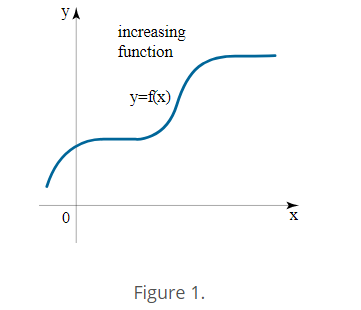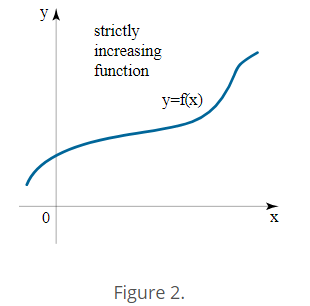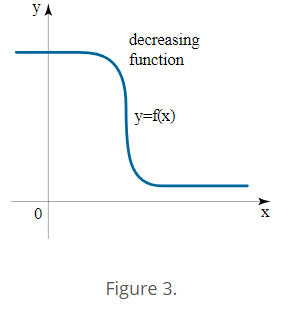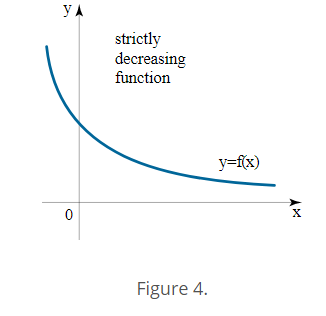If a function f(x) is differentiable on the interval (a,b) and belongs to one of the four considered types (i.e. it is increasing, strictly increasing, decreasing, or strictly decreasing), this function is called monotonic on this interval.

The concept of increasing and decreasing functions can also be defined for a single point x0. In this case, we consider a small δ-neighborhood (x0−δ,x0+δ) of this point. A function y=f(x) is strictly increasing at x0 if there exists a number δ>0 such that

∀x∈(x0−δ,x0)⇒f(x)<f(x0);

∀x∈(x0,x0+δ)⇒f(x)>f(x0).

Similarly, we can define a function y=f(x), which is strictly decreasing at the point x0.

Criteria for Increasing and Decreasing Functions

Again consider a function y=f(x) assuming it is differentiable on an interval (a,b). To determine if the function is increasing or decreasing on the interval, we use the sign of the first derivative of the function.

Theorem 1.
In order for the function y=f(x) to be increasing on the interval (a,b), it is necessary and sufficient that the first derivative of the function be non-negative everywhere in this interval:

f′(x)≥0∀x∈(a,b).

A similar criterion applies to the case of a function that is decreasing on the interval (a,b):

f′(x)≤0∀x∈(a,b).

We prove both (necessary and sufficient) parts of the theorem for the case of an increasing function.

Necessary condition.
Consider an arbitrary point x0∈(a,b). If the function y=f(x) is increasing on (a,b), then by definition, we can write:

∀x∈(a,b):x>x0⇒f(x)>f(x0);

∀x∈(a,b):x<x0⇒f(x)<f(x0).

It can be seen that in both cases the following inequality holds: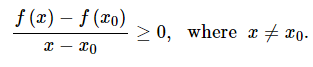In the limit as x→x0, the left-hand side of the inequality is equal to the derivative of the function at the point x0, that is by the limit sign preservation property: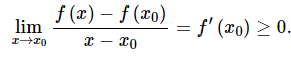This relation is valid for any x0∈(a,b).

Consider the sufficient condition, i.e. the converse statement. Suppose that the derivative f′(x) of a function y=f(x) is non-negative in the interval (a,b):

f′(x0)≥0∀x∈(a,b).

If x1 and x2 are two arbitrary points of the interval such that x1<x2, then by Lagrange’s theoremwe can write:

f(x2)−f(x1)=f′(c)(x2−x1),

where c∈[x1,x2], ⇒c∈(a,b).

Since f′(c)≥0, then the right-hand side of the equality is non-negative. Consequently,

f(x2)≥f(x1).

i.e. the function y=f(x) is increasing in the interval (a,b).

Consider now the cases of a strictly increasing and strictly decreasing function. There exists a similar theorem that describes the necessary and sufficient conditions. Omitting the proof, we state it for the case of a strictly increasing function.

Theorem 2.
Suppose that a function y=f(x) is differentiable on an interval (a,b). In order for the function to be strictly increasing in this interval, it is necessary and sufficient that the following conditions are satisfied:

1. f′(x)≥0∀x∈(a,b);

2. f′(x) is not identically equal to zero at any interval [x1,x2]∈(a,b).

The condition 1 is contained in Theorem 1 and is an indication of a non-decreasing function. The additional condition 2 is required in order to exclude the intervals of constancy, in which the derivative of f(x) is identically zero.

In practice (when finding the intervals of monotonicity), the sufficient condition for a strictly increasing or a strictly decreasing function is commonly used. Theorem 2 implies the following wording of the sufficient criterion:

If the condition f′(x)>0 is satisfied for all x∈(a,b), except perhaps only a few distinct points where f′(x)=0, then the function f(x) is strictly increasing in this interval.

Accordingly, the condition f′(x)<0 defines a strictly decreasing function.

The number of points where f′(x)=0 is usually finite. According to Theorem 2, they can not tightly fill any subinterval of the interval (a,b).

We also give a criterion for increasing/decreasing functions at a point:

Theorem 3.
Let x0∈(a,b).

• If f′(x0)>0, then the function f(x) is strictly increasing at the point x0;
• If f′(x0)<0, then the function f(x) is strictly decreasing at the point x0.

Properties of Monotonic Functions

Increasing and decreasing functions have certain algebraic properties, which may be useful in the investigation of functions. Here are some of them

1. If the functions f and g are increasing (decreasing) on the interval (a,b), then the sum of the functions f+g is also increasing (decreasing) on this interval

2. If the function f is increasing (decreasing) on the interval (a,b), then the opposite function −f is decreasing (increasing) on this interval.

3. If the function f is increasing (decreasing) on the interval (a,b), then the inverse function 1\f is decreasing (increasing) on this interval.

4. If the functions f and g are increasing (decreasing) on the interval (a,b) and moreover, f≥0, g≥0, then the product of the functions fg is also increasing (decreasing) on this interval.

5. If the function g is increasing (decreasing) on the interval (a,b) and the function f is increasing (decreasing) on (c,d) where g:(a,b)→(c,d), then the composition of functions f∘g

(i.e. the composite function y=f(g(x)) is also increasing (decreasing) on the interval (a,b).

Offer running on EduRev: Apply code STAYHOME200 to get INR 200 off on our premium plan EduRev Infinity!

197 docs

,

,

,

,

,

,

,

,

,

,

,

,

,

,

,

,

,

,

,

,

,

,

,

,

;